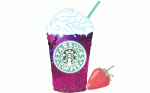Just For Fun Quiz / Desserts!!

Random Just For Fun Quiz

Can you name the Desserts!!?

bybethanycupcake  Plays Quiz not verified by Sporcle

Score
0/38
Timer
10:00
HintDessert
C_O_O_A_E C_K_
J_L_Y
O_E_S
R_C_ K_I_P_ T_E_T_
N_U_AT
F_D_E C_K_
F_U_T C_K_
U_S_D_W_ C_K_
D_V_L_ F_O_ C_K_
C_R_O_ C_K_
A_G_L C_K_
C_P_A_E_
B_N_N_ S_L_T
F_A_J_C_
M_D_I_A C_K_
S_O_T_R_A_
V_L_E_ C_K_
A_P_E P_E
R_C_ P_D_I_G
HintDessert
R_U_A_B C_U_B_E
M_R_N_U_
Y_L_ L_G
C_E_S_C_K_
B_N_U_Y C_K_
I_E C_E_M
W_T_R_E_O_
G_N_E_B_E_D
L_M_N C_K_
T_R_M_S_
T_I_L_
E_L_I_S
S_R_W_E_R_ C_E_S_C_K_
C_K_ P_P_
B_O_N_E
P_M_K_N P_E
Q_E_N_ P_D_I_G
H_Z_L_U_ C_K_
K_I_P_ K_E_M D_U_H_U_S

From the Vault See Another

50 Hits to Click: 1998

by caramba

Music 6m
Get those CDs back out of storage.

Extras

Created Jan 23, 2016
Tags:Dessert